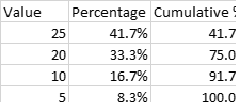# Creating a Column with the Cumulative Percentage

Hi, hope everyone is doing well,

I have a column with values, sorted in descending order, and I want to create a second column with the cumulative percentage of the first column’s total (end results would be the third column in the example below).This would be simple in Excel as I could just input the formula “=A2/SUM(\$A\$2:\$A\$5)” on the third column’s first row, “=(A3/SUM(\$A\$2:\$A\$5)) + 1” on the second row and so on. Another alternative would be having the formula “=IF(ROW(A2)=2;A2/SUM(\$A\$2:\$A\$5);(A2/SUM(\$A\$2:\$A\$5))+C1)” or something similar, therefore having a single formula.

I could use the Math Formula node to do the \$column\$/COL_SUM(\$column\$), which would get me the percentage of that specific row, but I don’t know how to reference row/cell position for the cumulative sum.

Is there any way to do this? Additionally, any recommendations of reading material for KNIME formula’s syntax would be really helpful, I’m a bit of an Excel rat, I guess KNIME will take some getting used to!

Thanks a mill

Hi there!

Sure there is a way! For calculating cumulative sum you can use multiple nodes. Try Moving Aggregation node with Cumulative computation checked and and Aggregation method on wanted column to be sumYou can also use Java Snippet (simple) node if you prefer codingIf you are coming from Excel check this out:

https://www.knime.com/knimepress/from-excel-to-knime

Br,
Ivan

4 Likes

Worked perfectly, cheers! And thanks for the tip!

2 Likes

You are welcomeIvan

1 Like

This topic was automatically closed 7 days after the last reply. New replies are no longer allowed.